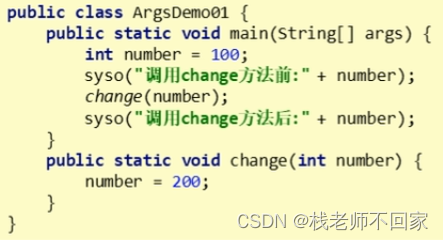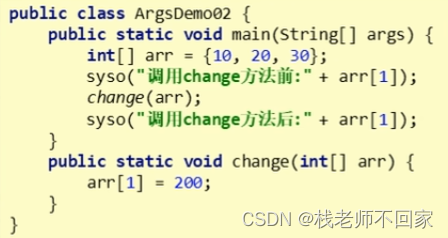# Java 方法

### 文章目录

• 1. 方法的定义和调用
• 2. 带参方法的定义和调用
• 3. 带返回值方法的定义和调用
• 4. 方法的注意事项
• 5. 方法重载
• 6. 方法的参数传递

## 1. 方法的定义和调用

① 方法必须先创建才可以使用，该过程称为方法定义；
② 方法创建后并不是直接运行的，需要手动使用后才会执行，该过程称为方法调用。

① 方法定义

``````public static void isTest() {
//方法体
}
``````

② 方法调用

``````isTest();
``````
``````//定义一个方法，在方法中定义一个变量，判断该数据是否是偶数
public class MethodDemo {public static void main(String[] args) {isTest();}public static void isTest() {int number = 10;if(number % 2 == 0) {System.out.println(true);} else {System.out.println(false);}}
}
``````

`在 main 方法中调用自定义方法！`

## 2. 带参方法的定义和调用

① 方法定义

``````//单个参数方法的定义
public static void isTest(int num) {}
//多个参数方法的定义
public static void isTest(int num1, int num2) {}
``````

`多个参数之间用逗号隔开！`

② 方法调用

``````//单个参数方法的调用
isTest(5);
//多个参数方法的调用
isTest(5, 6);
``````

`方法调用时，参数的数量与类型必须与方法定义中的设置相匹配，否则程序将报错！`

``````public class MethodDemo {public static void main(String[] args) {int number = 10;isTest(number);}public static void isTest(int num) {if(num % 2 == 0) {System.out.println(true);} else {System.out.println(false);}}
}
``````

① 形参是方法定义中的参数，形式上的参数，如上的 num；
② 实参是方法调用中的参数，实际的参数，如上的 number。

## 3. 带返回值方法的定义和调用

① 方法定义

``````public static int getMax(int a, int b) {...return c;
}
``````

`注意方法定义中的数据类型，要与返回值的数据类型保持一致！`

② 方法调用

``````int max = getMax(7, 9);
``````

`方法的返回值通常会使用变量来接收，否则该返回值将无意义！`

``````public class MethodDemo {public static void main(String[] args) {boolean flag = isEvenNumber(10);System.out.println(flag);}public static boolean isEvenNumber(int number) {if(number % 2 == 0) {return true;} else {return false;}}
}
``````

## 4. 方法的注意事项

① 方法之间是平级关系，不能嵌套定义；
② void 表示无返回值，可以省略 return，也可以单独地书写 return，但是后面不允许加数据；
③ return 会终止语句，return 之后的代码不再执行；
④ 定义方法时，要做到两个明确，第一个就是明确返回值类型，主要是明确方法操作完毕之后是否有数据返回，如果没有写 void，如果有写对应的数据类型，第二个要明确参数，主要是明确参数的类型和数量；
⑤ 调用方法时，void 类型的方法直接调用即可，非 void 类型的方法推荐用变量接收调用。

## 5. 方法重载

① 多个方法在同一个类中
② 多个方法具有相同的方法名
③ 多个方法的参数不相同，类型不同或者数量不同。

① 重载仅对应方法的定义，与方法的调用无关，调用方式参照标准格式；
② 重载仅针对同一个类中方法的名称与参数进行识别，与返回值无关，换句话说不能通过返回值来判定两个方法是否构成重载。

``````public class MethodDemo {public static void main(String[] args) {int result1 = sum(10, 20);System.out.println(result1);double result2 = sum(10.0, 20.0);System.out.println(result2);}public static int sum(int a, int b) {return a + b;    }public static double sum(double a, double b) {return a + b;}
}
``````

`方法重载与返回值无关，Java 虚拟机会通过参数的不同来区分同名的方法！`

## 6. 方法的参数传递

① 基本类型参数传递`对于基本数据类型的参数，形式参数的改变，不会影响实际参数的值！`

②引用类型参数传递`对于引用类型的参数，形式参数的改变，会影响实际参数的值，而数组属于引用类型参数，所以会受到影响！`